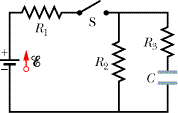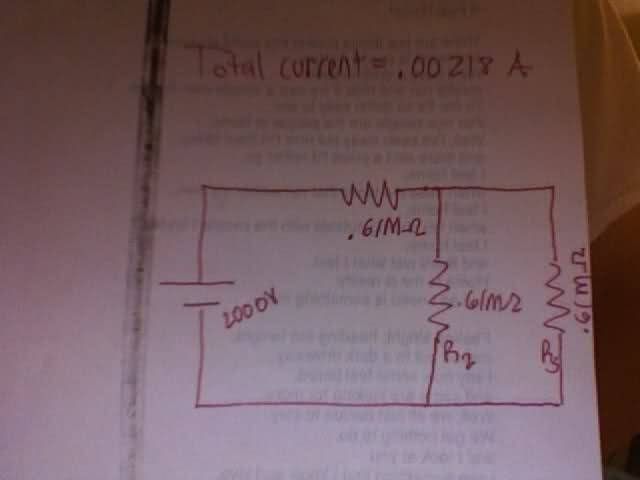# RC Circuit/Current/Resistance Problem. Circuit Gurus come here

• Divide
In summary: What does the equivalent circuit look like? What did you get for the total current. What did you get for the voltage across R1? And so the...

## Homework Statement

In the circuit of the figure below, script e = 2.0 kV, C = 7.0 µF, R1 = R2 = R3 = 0.61 MΩ. With C completely uncharged, switch S is suddenly closed (at t = 0).(a) At t = 0, what is current i1 in resistor 1?
(b) At t = 0, what is current i2 in resistor 2?
(c) At t = 0, what is current i3 in resistor 3?

Repeat for t = infinity (that is, after many time constants.)
(d) What is current i1?
(e) What is current i2?
(f) What is current i3?
(g) What is the potential difference V2 across resistor 2 at t = 0?
7 V
(h) What is V2 at t = infinity?

## Homework Equations

V=IR I=Change(Q)/Change(T)
Series Wiring=Rs=R1+R2+...

RC Circuit: q=q0[1-e^-t/(RC)]-Charge in capacitor.
T=RC
Discharge of Capacitor-q=q0e^-t/(RC)

## The Attempt at a Solution

I've been trying this for days and have a test on it tomorrow. I have the faintest idea where to start. Thanks for the help in advance, I need it badly.

Divide said:

## Homework Statement

In the circuit of the figure below, script e = 2.0 kV, C = 7.0 µF, R1 = R2 = R3 = 0.61 MΩ. With C completely uncharged, switch S is suddenly closed (at t = 0).(a) At t = 0, what is current i1 in resistor 1?
(b) At t = 0, what is current i2 in resistor 2?
(c) At t = 0, what is current i3 in resistor 3?

Repeat for t = infinity (that is, after many time constants.)
(d) What is current i1?
(e) What is current i2?
(f) What is current i3?
(g) What is the potential difference V2 across resistor 2 at t = 0?
7 V
(h) What is V2 at t = infinity?

## Homework Equations

V=IR I=Change(Q)/Change(T)
Series Wiring=Rs=R1+R2+...

RC Circuit: q=q0[1-e^-t/(RC)]-Charge in capacitor.
T=RC
Discharge of Capacitor-q=q0e^-t/(RC)

## The Attempt at a Solution

I've been trying this for days and have a test on it tomorrow. I have the faintest idea where to start. Thanks for the help in advance, I need it badly.

AT t=0, the capacitor is uncharged so treat the capacitor as if it was just a wire. Then you just have a simple circuit with resistors only.

At t=infinity, there is no current in the section containing the capacitor (so no current in R3)
You just have a simple circuit with the battery connected with R1 and R2 in series.
The voltage across the capacitor is the same as the voltage across R2

Thanks a bunch dude, but what does Mohm mean?

Welcome to PF!

Hi Divide! Welcome to PF!Divide said:
Thanks a bunch dude, but what does Mohm mean?

Mega.1 Mega Ohm = 1 * 10^6 Ohms.

Thanks guys, but I'm still kinda confused. What would i do to get the current at R1 R2 and R3?

Divide said:
Thanks guys, but I'm still kinda confused. What would i do to get the current at R1 R2 and R3?

nrqed gave you the hints in post #2. Show us your attempt at a solution based on those hints.

Well for resistor 1 I tried 2000V/610000ohm to get the current, but that's apparently wrong. Would I use Kirchhoff's laws?

Divide said:
Well for resistor 1 I tried 2000V/610000ohm to get the current, but that's apparently wrong. Would I use Kirchhoff's laws?

You would use series and parallel combinations of resistors to calculate current flows. As nrqed hinted, when the switch is first closed, the capacitor acts like a short circuit. So re-draw the circuit using that hint for the first set of questions.

For t = infinity, the voltages have all reached equilibrium, and there is no current flow through the capacitor (looks like an open circuit). Re-draw the circuit for this case, and answer the second set of questions.

berkeman said:
You would use series and parallel combinations of resistors to calculate current flows. As nrqed hinted, when the switch is first closed, the capacitor acts like a short circuit. So re-draw the circuit using that hint for the first set of questions.

For t = infinity, the voltages have all reached equilibrium, and there is no current flow through the capacitor (looks like an open circuit). Re-draw the circuit for this case, and answer the second set of questions.

Ok thanks, I drew the equivalent circuit for part 1 and got the total current. I then got the voltage across R1. But I'm having loads of trouble finding the voltage across the two parallel resistors. Any suggestions?

Divide said:
Ok thanks, I drew the equivalent circuit for part 1 and got the total current. I then got the voltage across R1. But I'm having loads of trouble finding the voltage across the two parallel resistors. Any suggestions?

What does the equivalent circuit look like? What did you get for the total current. What did you get for the voltage across R1? And so the voltage across the parallel resistors would be... what?That's the complete circuit.
The total current is .00218

The voltage across R1 is 1322.85V

I don't know what to do with the parallel resistors.

The equivalent resistance of the two parallel resistors is .305Mohm

Divide said:That's the complete circuit.
The total current is .00218

The voltage across R1 is 1322.85V

I don't know what to do with the parallel resistors.

How did you get the total current and V(R1)? You would have had to deal with the parallel resistors to get those answers, right? What do the voltage drops have to total up to equal when you add up all the drops around a closed loop?

berkeman said:
How did you get the total current and V(R1)? You would have had to deal with the parallel resistors to get those answers, right? What do the voltage drops have to total up to equal when you add up all the drops around a closed loop?

I know the voltage drops add up to 2kV. I got the equivalent resistance of the two parallel resistors as .305Mohms. I got the todal resistance by finding the equivalent resistance of the entire circuit by adding the resistors in series and parallel. Then to get the total current I used ohm's law(V=IR) to get the total current.

I know the current at R1 has to be the same as the total current, but I am having serious trouble finding the current between the two separate parallel resistors.

Divide said:
I know the voltage drops add up to 2kV. I got the equivalent resistance of the two parallel resistors as .305Mohms. I got the todal resistance by finding the equivalent resistance of the entire circuit by adding the resistors in series and parallel. Then to get the total current I used ohm's law(V=IR) to get the total current.

I know the current at R1 has to be the same as the total current, but I am having serious trouble finding the current between the two separate parallel resistors.

The voltage drop across the two parallel resistors is just what is left over after you subtract V(R1) from the source voltage of 2kV. The voltage is the same across both of them. Then, since you have the voltage across them, you can calculate the current through each if needed. Make sense?

berkeman said:
The voltage drop across the two parallel resistors is just what is left over after you subtract V(R1) from the source voltage of 2kV. The voltage is the same across both of them. Then, since you have the voltage across them, you can calculate the current through each if needed. Make sense?

Thanks so much! Yeah I had all the numbers i just wasn't doing the math right. Let's hope i can do this for the T=infinity lol.